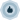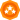Choose a Product Community
Resources

## How to access a variable outside a method in groovy ?

SOLVEDFrequent Contributor

## How to access a variable outside a method in groovy ?

Hi,

i have a variable at project leve like: \${#Project#value}

i have a groovy step with this:

```//VAR
//def expected_value = testRunner.testCase.testSuite.project.getPropertyValue("value") as Integer

//TEST
def test_validate_name(){
def responseContent = testRunner.testCase.testSuite.project.getPropertyValue( "response" )
def slurperresponse = new JsonSlurper().parseText(responseContent)

float expected_value = 200
float[] a = slurperresponse.values.DATA.FILE..value
float actual_value  = a.collect { "\$it" }.join( '' ) as float
assert actual_value <= expected_value
}```

It works if i keep this line inside the medthod:

float expected_value = 200

If i put comment on this line and use the variable from outside the method with this line:

def expected_value = testRunner.testCase.testSuite.project.getPropertyValue("value") as Integer

it throws error: java.lang.NullPointerException: Cannot invoke method test_validate_name() on null object

I tried with this set.context('{Project#value}') it doesn't work.

Do you have any suggestions ?

21 REPLIES 21Champion Level 0

If I am not mistaken I am not sure that you can reference the variable OUTSIDE the method INSIDE the method without passing it in as a parameter.

---

Click the Accept as Solution button if my answer has helped, and remember to give kudos where appropriate too!Champion Level 3

Couple of things:

1. If that is the whole script, I could not see the need for the method.

2. If method is needed, assuming that the method is called more than once in this script step

3. the value is not accesssible because of the scope

- a. either pass the value to the method

say, def method(expectedValue) {...} and use method(variable)

- b. compute the value from inside the method like you created responseContent

Regards,
Rao.Frequent Contributor

1. If that is the whole script, I could not see the need for the method:

No it's not the whole script. This script is called from another class.Champion Level 3

Regards,
Rao.Frequent Contributor

if i get answer i will post here.

i did not figure out yet.

i was thinking about using context.expand for the variable.Champion Level 3

```def test_validate_name(){
def responseContent = testRunner.testCase.testSuite.project.getPropertyValue( "response" )
def slurperresponse = new JsonSlurper().parseText(responseContent)
//You can use as below
float expected_value = testRunner.testCase.testSuite.project.getPropertyValue("value")
float[] a = slurperresponse.values.DATA.FILE..value
float actual_value  = a.collect { "\$it" }.join( '' ) as float
assert actual_value <= expected_value
}```

Regards,
Rao.Frequent Contributor

Hi,

i understand this and im using it actually.

suppose we have 2 methods:

def test_validate_name()

I need to validate with the same variable expected_value

How do you delcare the variable?

The variable expected_value should be accessible everywhere, especially in these 2 methods.

This avoid the repetition of the following 3 lines in each methods.

```float expected_value = testRunner.testCase.testSuite.project.getPropertyValue("value")
float[] a = slurperresponse.values.DATA.FILE..value
float actual_value = a.collect { "\$it" }.join( '' ) as float```

The first method:

```def test_validate_name(){
def responseContent = testRunner.testCase.testSuite.project.getPropertyValue( "response" )
def slurperresponse = new JsonSlurper().parseText(responseContent)
//You can use as below
float expected_value = testRunner.testCase.testSuite.project.getPropertyValue("value")
float[] a = slurperresponse.values.DATA.FILE..value
float actual_value  = a.collect { "\$it" }.join( '' ) as float
assert actual_value <= expected_value
}```

I hope i'm clear.Champion Level 3

Not sure If missed something.

Isn't this you wanted?

```float expected_value = testRunner.testCase.testSuite.project.getPropertyValue("value")

def test_validate_name(expected){
def responseContent = testRunner.testCase.testSuite.project.getPropertyValue( "response" )
def slurperresponse = new JsonSlurper().parseText(responseContent)
float[] a = slurperresponse.values.DATA.FILE..value
float actual_value  = a.collect { "\$it" }.join( '' ) as float
//Using below the passed value
assert actual_value <= expected
}

test_validate_name(expected_value)```

Regards,
Rao.Frequent Contributor

We are almost there but it's not working for me.

i modified these lines

`def test_validate_name(expected) to def test_validate_name(expected_value)`
`assert actual_value <= expected to assert actual_value <= expected_value`

But when i call from another class from another testsuite it says it cannot find expected_value

It works if i put this line

`float expected_value = testRunner.testCase.testSuite.project.getPropertyValue("value")`

inside the method

`def test_validate_name()`

Can we just use def expected_value = context.expand('Project#value')

i don't know how to call this variable inside the method like:

`assert actual_value <= expected_value `

Also i saw this in google:

```import groovy.transform.Fieldvar1 = 'var1'
@Field String var2 = 'var2'
def var3 = 'var3'
void printVars() {    println var1
println var2
println var3 // This won't work, because not in script scope.
}```cancel
Showing results for